# The Sphenomegacorona

The sphenomegacorona is the 88th Johnson solid (J88). Its surface consists of 16 equilateral triangles and 2 squares, for a total of 18 faces, 28 edges, and 12 vertices.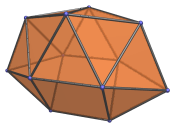The sphenomegacorona is one of the special Johnson solids at the end of Norman Johnson's list that are not directly derived from the uniform polyhedra by cut-and-paste operations. As Norman Johnson explains, a lune is a square with two opposite edges attached to equilateral triangles, and a spheno (Latin for wedge) complex is two lunes joined together to form a wedge-like structure. A corona is a crown-like complex of 8 triangles, whereas a megacorona is a larger corona with 12 triangles. Sphenomegacorona, therefore, describes the structure built from a spheno complex attached to a megacorona of 12 triangles.

## Projections

Here are some views of the sphenomegacorona from various angles:

Projection Description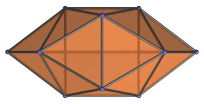Top view.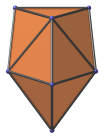Front view.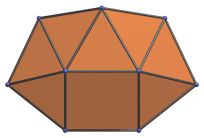Side view.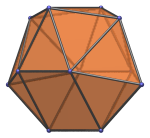45° side view.

## Coordinates

The Cartesian coordinates of the sphenomegacorona with edge length 2 are:

• (±1, 0, 2B)
• (±1, ±2A, 0)
• (±(C+B)/B, 0, D/B)
• (0, ±1, −√(2+4A−4A2))
• (±(1+CD/B3), 0, (2A4−1)/B3)

where:

• B = √(1−A2)
• C = √(3−4A2)
• D = 1−2A2

and A is the root of the following polynomial between 0.5 and 0.6:

1680A16 − 4800A15 − 3712A14 + 17216A13 + 1568A12 − 24576A11 + 2464A10 + 17248A9 − 3384A8 − 5584A7 + 2000A6 + 240A5 − 776A4 + 304A3 + 200A2 − 56A − 23 = 0

The approximate value of A is 0.594633335632639.

## Credits

The above coordinates were adapted from the solution published by A. V. Timofeenko (2008), The non-Platonic and non-Archimedean noncomposite polyhedra, Fundamentalnaya i Prikladnaya Matematika, vol.14 (2), pp.179-205. (А. В. Тимофеенко (2008) «Несоставные многогранники, отличные от тел Платона и Архимеда», Фундаментальная и Прикладная Математика, том 14-й (2), ст.179-205.)

Last updated 18 Jun 2019.